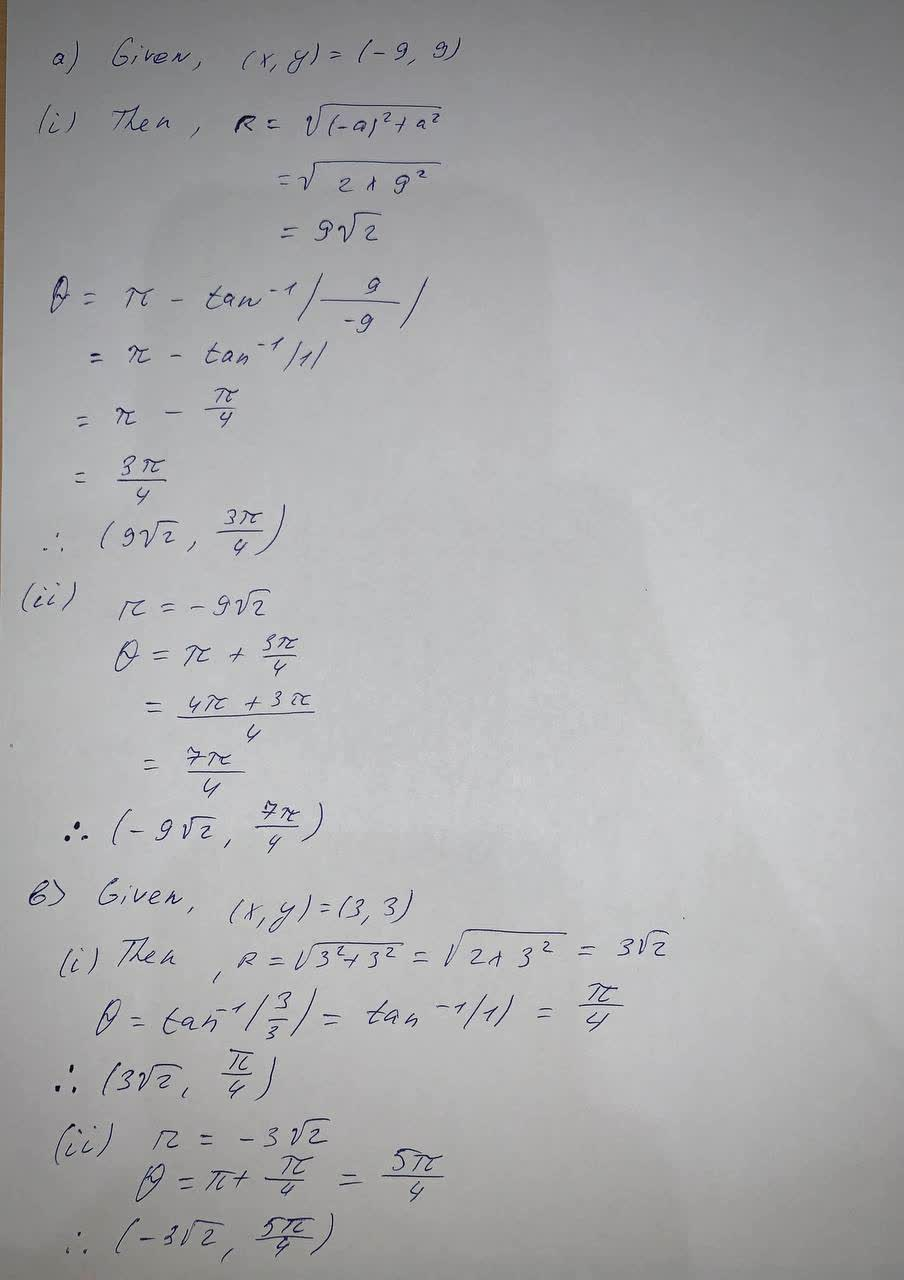Question# The Cartesian coordinates of a point are given.(-9, 9) (i) Find polar coordinates (r, theta)of the point, where r > 0

Parametric equations
ANSWEREDThe Cartesian coordinates of a point are given.
(−9, 9)
(i) Find polar coordinates $$(r, \theta)$$ of the point, where r > 0
and $$\displaystyle{0}≤\theta{<}{2}?.$$